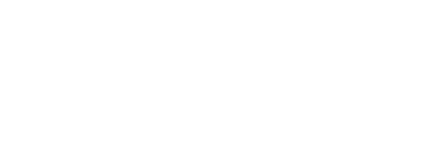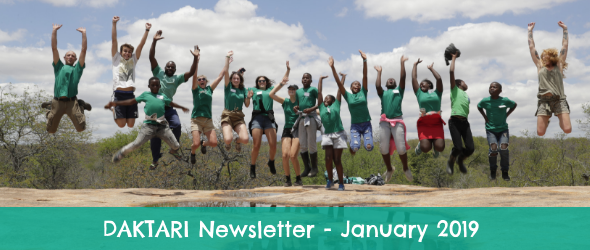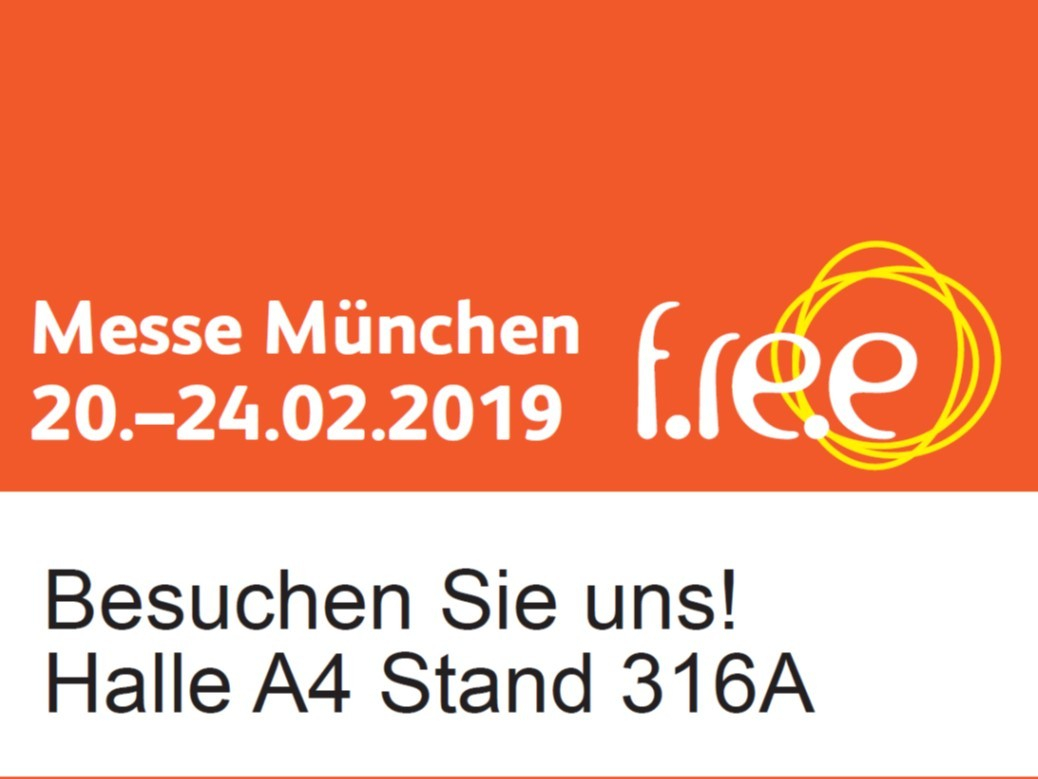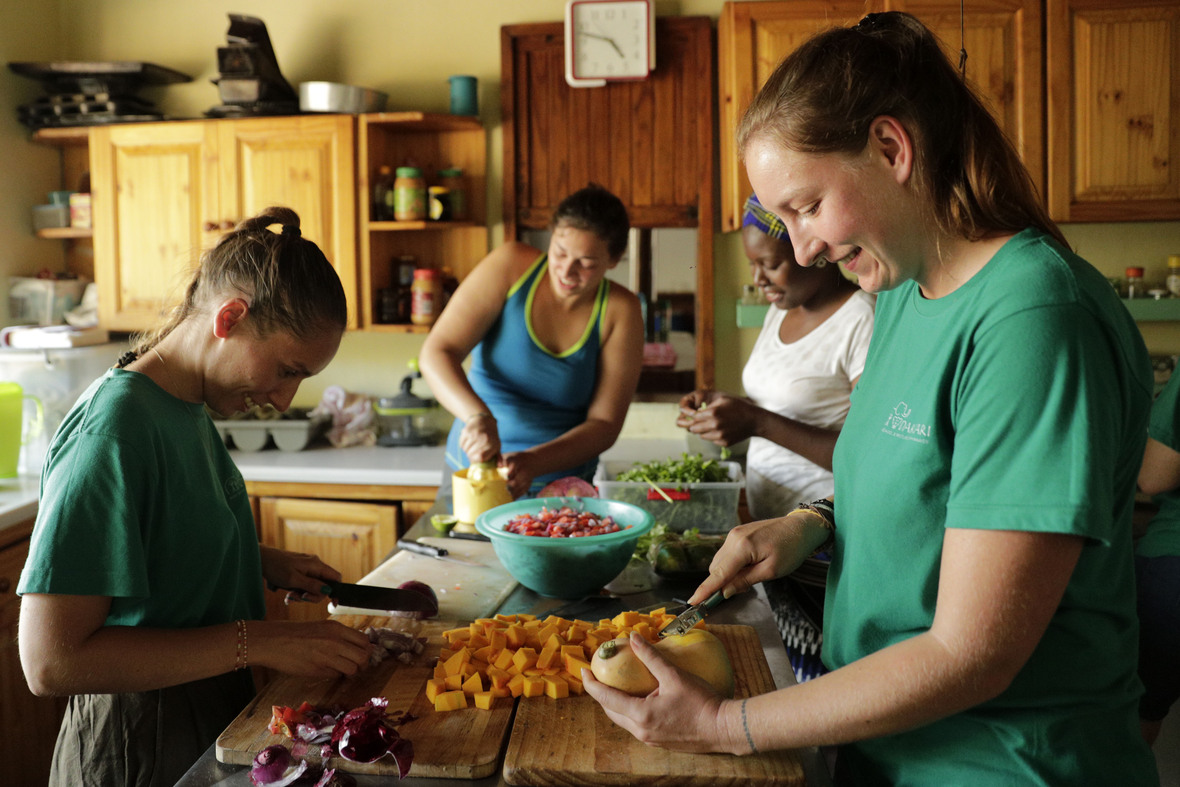/* styles */ Welcome in our first newsletter of the year!

 table div table+table+table+table div table,table.module-3{width:66.42%;float:right;padding:0}table div table+table+table+table div table a{border:0 none;text-decoration:none}table div table+table+table+table div table img{width:100%!important;border:0 none;text-decoration:none}table div table+table+table+table div table td{width:100%;padding:0 0 20px 20px}/* styles */ We opened a new project on GlobalGiving for our Job Hunting Program. By supporting more participants in our Job Hunting Program you will help us improve their future!
 /* styles */ During our Job Hunting Program we welcome young adult students to join us for a week of learning. With a 50% unemployment rate for newly graduated youth, this is an important program. The skills taught include writing a CV and cover letter, setting up an email address and basic computer skills.
 table div table+table+table+table+table+table div table{width:100%;padding:0}table div table+table+table+table+table+table div table img{width:96.23%;padding:0;float:none}table div table+table+table+table+table+table div table td{width:100%;padding:0 1.88% 18px}/* styles */## Interview with Rahaba

 /* styles */ Rahaba, our Outreach Assistant, was interviewd by GoAbroad.com about her job! Click on her picture to read her interesting interview!
 table.module-8{width:49.81%;padding:0}table div table+table+table+table+table+table+table+table+table div table{width:49.81%;float:none;margin-left:auto;margin-right:auto;padding:0}table div table+table+table+table+table+table+table+table+table div table a{border:0 none;text-decoration:none}table div table+table+table+table+table+table+table+table+table div table img{width:100%!important;border:0 none;text-decoration:none}table div table+table+table+table+table+table+table+table+table div table td{width:100%;padding:0}/* styles */
 table div table+table+table+table+table+table+table+table+table+table div table{width:100%;padding:0}table div table+table+table+table+table+table+table+table+table+table div table img{width:96.23%;padding:0;float:none}table div table+table+table+table+table+table+table+table+table+table div table td{width:100%;padding:0 1.88% 18px}/* styles */## FREE Munich 2019If you are in Munich between the 20th and 24th of February, do not hesitate to come and meet DAKTARI on the FREE trade show!

 table div table+table+table+table+table+table+table+table+table+table+table+table+table div table{width:100%;padding:0}table div table+table+table+table+table+table+table+table+table+table+table+table+table div table img{width:96.23%;padding:0;float:none}table div table+table+table+table+table+table+table+table+table+table+table+table+table div table td{width:100%;padding:0 1.88% 18px}/* styles */## Our kitchen renovation is about to start

 /* styles */ After three months of fundraising to renovate our old kitchen, the builders are arriving the 3rd of February to step into ACTION! We thanks, so far: * Wilma & Koen Barend who triggered the project with a generous donation to encourage us * All our individual donors who donated through our GlobalGiving platform * Electrolux * Hirsh's * Sephaku cement * Stonebuilt * Buco, our main partner for this project, for their assistance and guidance. Progress will be on our facebook page, so stay tuned to be updated!table div table+table+table+table+table+table+table+table+table+table+table+table+table+table+table+table+table div table{width:100%;padding:0}table div table+table+table+table+table+table+table+table+table+table+table+table+table+table+table+table+table div table img{width:96.23%;padding:0;float:none}table div table+table+table+table+table+table+table+table+table+table+table+table+table+table+table+table+table div table td{width:100%;padding:0 1.88% 18px}/* styles *//* styles */ Check out our blog to stay up to date with all news! Thinking about volunteering? Visit our website to see all the opportunities
 table.module-18{width:22.08%;padding:0}table div table+table+table+table+table+table+table+table+table+table+table+table+table+table+table+table+table+table+table div table{width:22.08%;float:none;margin-left:auto;margin-right:auto;padding:0}table div table+table+table+table+table+table+table+table+table+table+table+table+table+table+table+table+table+table+table div table a{border:0 none;text-decoration:none}table div table+table+table+table+table+table+table+table+table+table+table+table+table+table+table+table+table+table+table div table img{width:100%!important;border:0 none;text-decoration:none}table div table+table+table+table+table+table+table+table+table+table+table+table+table+table+table+table+table+table+table div table td{width:100%;padding:0}/* styles */
 table.module-19{width:22.26%;padding:0}table div table+table+table+table+table+table+table+table+table+table+table+table+table+table+table+table+table+table+table+table div table{width:22.26%;float:none;margin-left:auto;margin-right:auto;padding:0}table div table+table+table+table+table+table+table+table+table+table+table+table+table+table+table+table+table+table+table+table div table a{border:0 none;text-decoration:none}table div table+table+table+table+table+table+table+table+table+table+table+table+table+table+table+table+table+table+table+table div table img{width:100%!important;border:0 none;text-decoration:none}table div table+table+table+table+table+table+table+table+table+table+table+table+table+table+table+table+table+table+table+table div table td{width:100%;padding:0}/* styles */
 Like   Tweet   Pin   +1   in